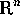Homework Problems for Chapter 2
The Vector Space• Problems marked with an asterisk () will be collected and graded. In general, problems will be due the day following the lecture in which the section was discussed.
• The selected problems require a mixture of computation and theory to answer. In most cases the problems are not difficult once the question is identified and understood. The key to this is a thorough understanding of the vocabulary and various connections between the concepts.
• If you need more practice, there are many other problems in the text that are similar to the ones I have assigned.
• There are two sections of review problems at the end of each chapter. None of these problems will be collected for grading. These problems should be a good test of your understanding of the main points in the chapter. I suggest solving these problems as one part of your preparation for an exam.
• Be sure you know how to do all of these problems. Do not hesitate to ask questions - in class, by e-mail, or during office hours.